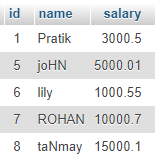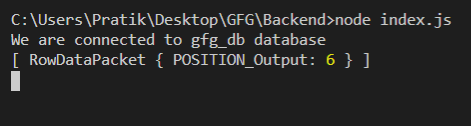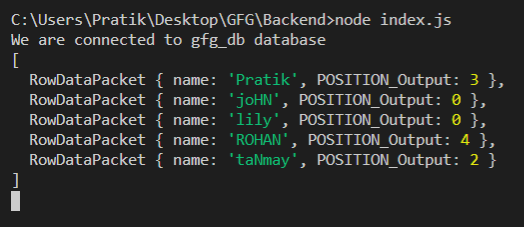Related Articles
NodeJS MySQL POSITION() Function
• Last Updated : 18 Feb, 2021

POSITION() Function is a Builtin Function in MySQL which is used to get position of first occurrence of a pattern in a text when searched from specific position.

Note: It is not Case Sensitive.

Syntax:

`POSITION(pattern IN text)`

Parameters: POSITION() function accepts two parameters as mentioned above and described below.

• pattern: Pattern to be Searched
• text: In this text, pattern will be searched

Return Value: POSITION() function returns position of first occurrence of a pattern in a text when searched from specific position. If something went wrong it will return 0.

Modules:

• mysql: To handle MySQL Connection and Queries
`npm install mysql`

SQL publishers Table Preview:Example 1:

## Javascript

 `const mysql = require(``"mysql"``);`` ` `let db_con = mysql.createConnection({``  ``host: ``"localhost"``,``  ``user: ``"root"``,``  ``password: ``""``,``  ``database: ``"gfg_db"``,``});`` ` `db_con.connect((err) => {``  ``if` `(err) {``    ``console.log(``"Database Connection Failed !!!"``, err);``    ``return``;``  ``}`` ` `  ``console.log(``"We are connected to gfg_db database"``);`` ` `  ``// notice the ? in query``  ``let query = `SELECT POSITION(``'for'` `IN ``'GEEKSFORGEEKS'``) AS POSITION_Output`;`` ` `  ``db_con.query(query, (err, rows) => {``    ``if` `(err) ``throw` `err;`` ` `    ``console.log(rows);``  ``});``});`

Output:Example 2:

## Javascript

 `const mysql = require(``"mysql"``);`` ` `let db_con = mysql.createConnection({``  ``host: ``"localhost"``,``  ``user: ``"root"``,``  ``password: ``""``,``  ``database: ``"gfg_db"``,``});`` ` `db_con.connect((err) => {``  ``if` `(err) {``    ``console.log(``"Database Connection Failed !!!"``, err);``    ``return``;``  ``}`` ` `  ``console.log(``"We are connected to gfg_db database"``);`` ` `  ``// notice the ? in query``  ``let query = `SELECT name, POSITION(``'a'` `IN name) ``               ``AS POSITION_Output FROM publishers`;`` ` `  ``db_con.query(query, (err, rows) => {``    ``if` `(err) ``throw` `err;`` ` `    ``console.log(rows);``  ``});``});`

Output:My Personal Notes arrow_drop_up